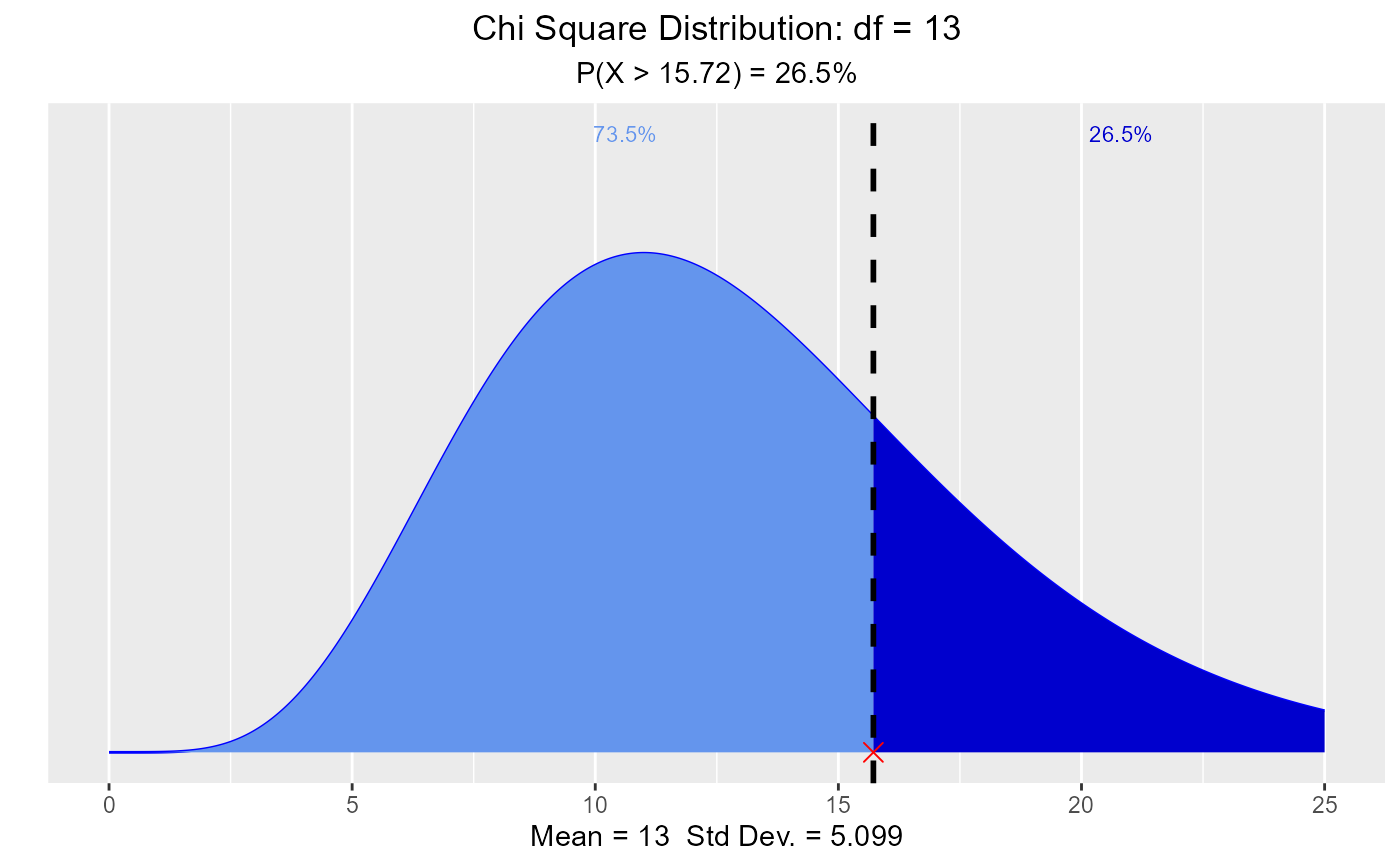Visualize how changes in degrees of freedom affect the shape of the chi square distribution. Compute & visualize quantiles out of given probability and probability from a given quantile.

vdist_chisquare_plot(
df = 3,
normal = FALSE,
xaxis_range = 25,
print_plot = TRUE
)

vdist_chisquare_perc(
probs = 0.95,
df = 3,
type = c("lower", "upper"),
print_plot = TRUE
)

vdist_chisquare_prob(
perc = 13,
df = 11,
type = c("lower", "upper"),
print_plot = TRUE
)

## Arguments

df Degrees of freedom. If TRUE, normal curve with same mean and sd as the chi square distribution is drawn. The upper range of the X axis. logical; if TRUE, prints the plot else returns a plot object. Probability value. Lower tail or upper tail. Quantile value.

Chisquare

## Examples

# visualize chi square distribution
vdist_chisquare_plot()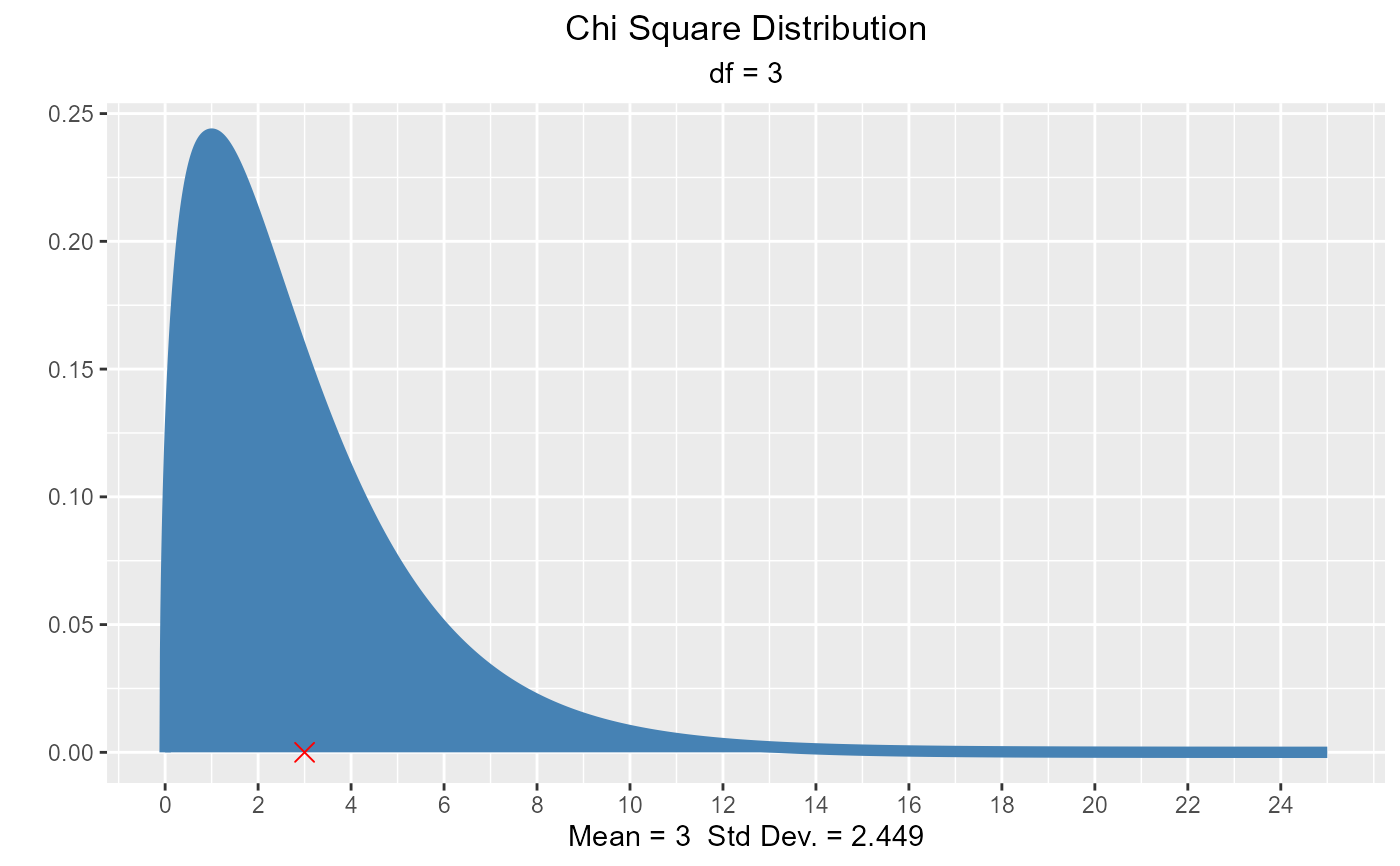vdist_chisquare_plot(df = 5)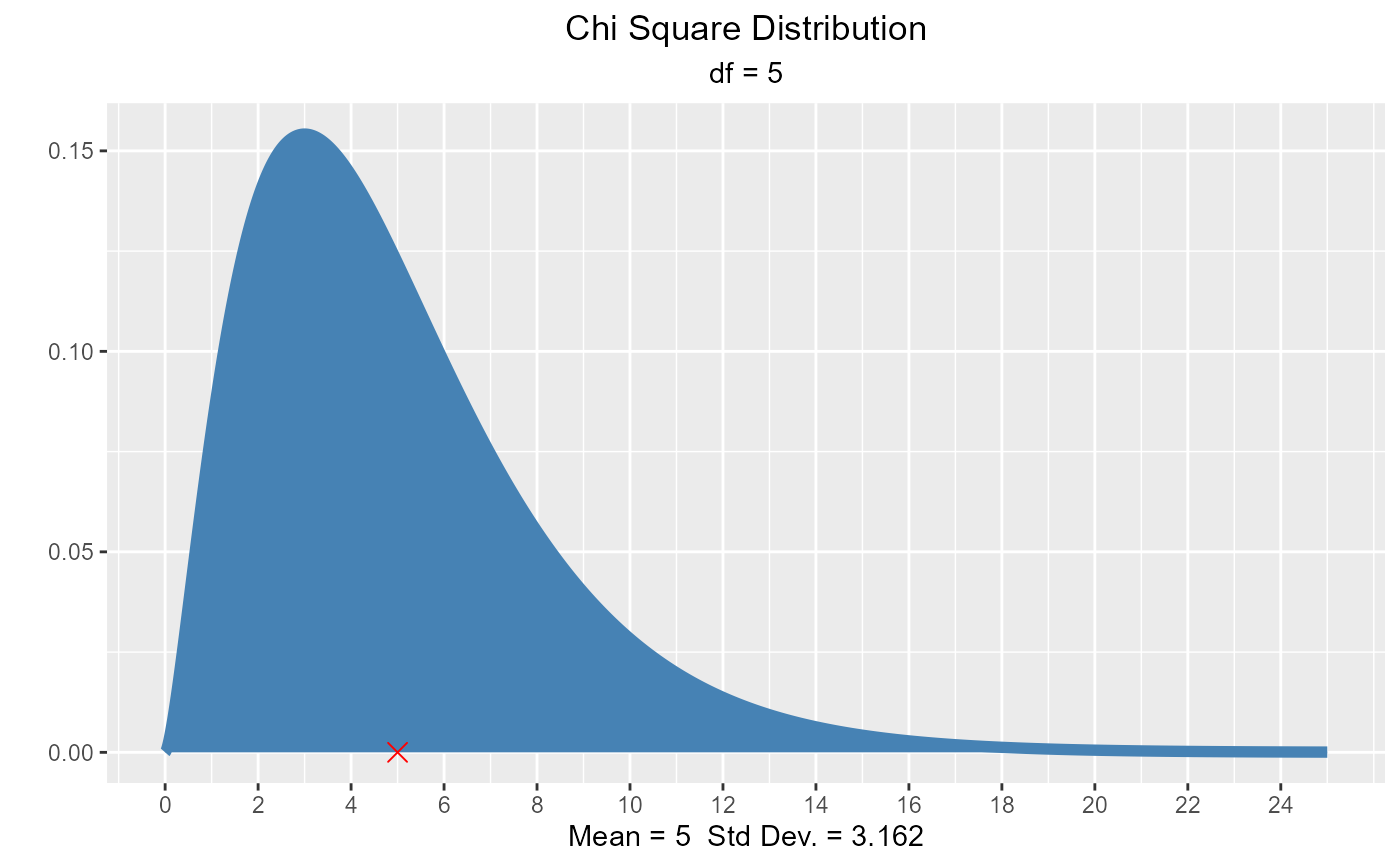vdist_chisquare_plot(df = 5, normal = TRUE)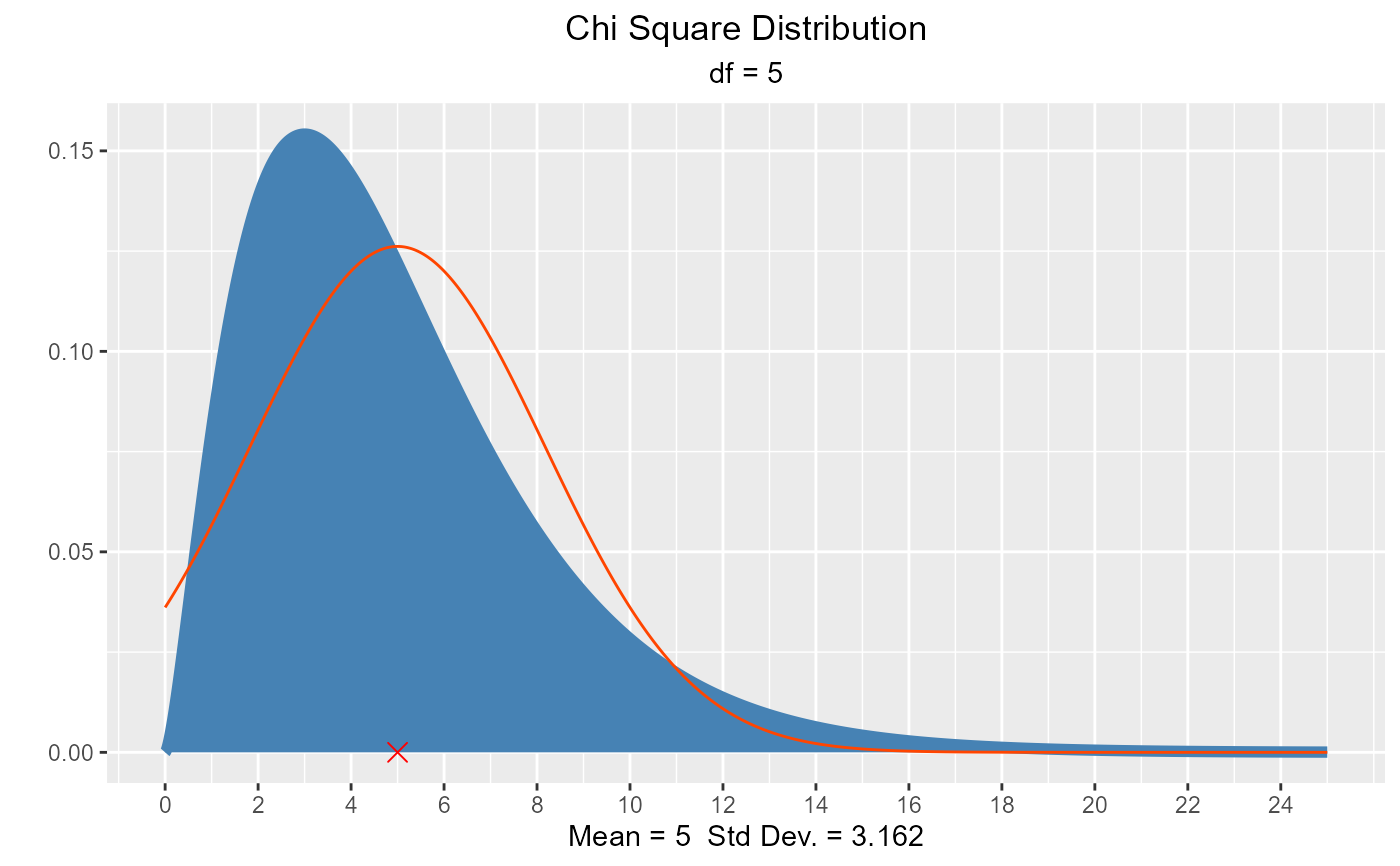# visualize quantiles out of given probability
vdist_chisquare_perc(0.165, 8, 'lower')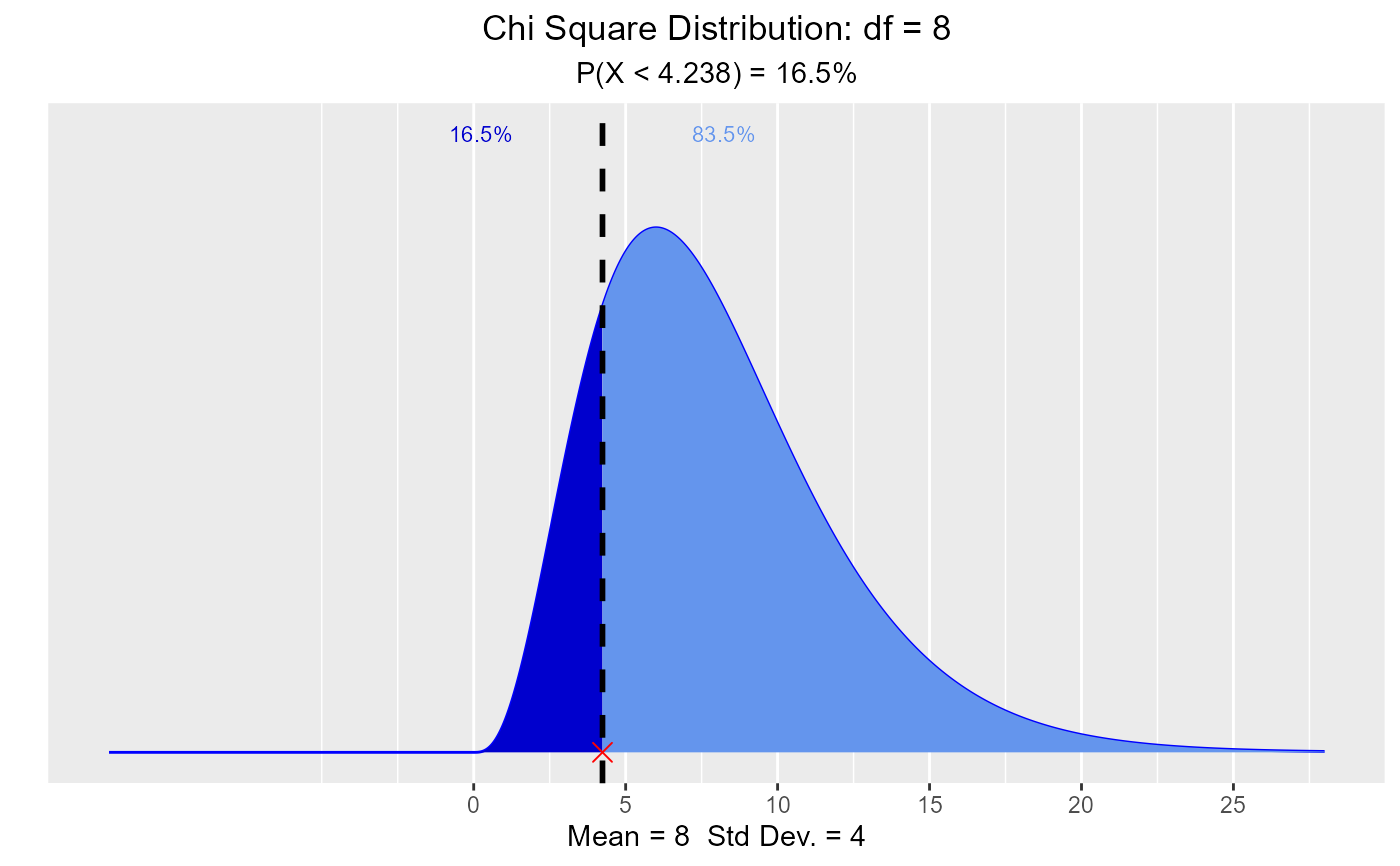vdist_chisquare_perc(0.22, 13, 'upper')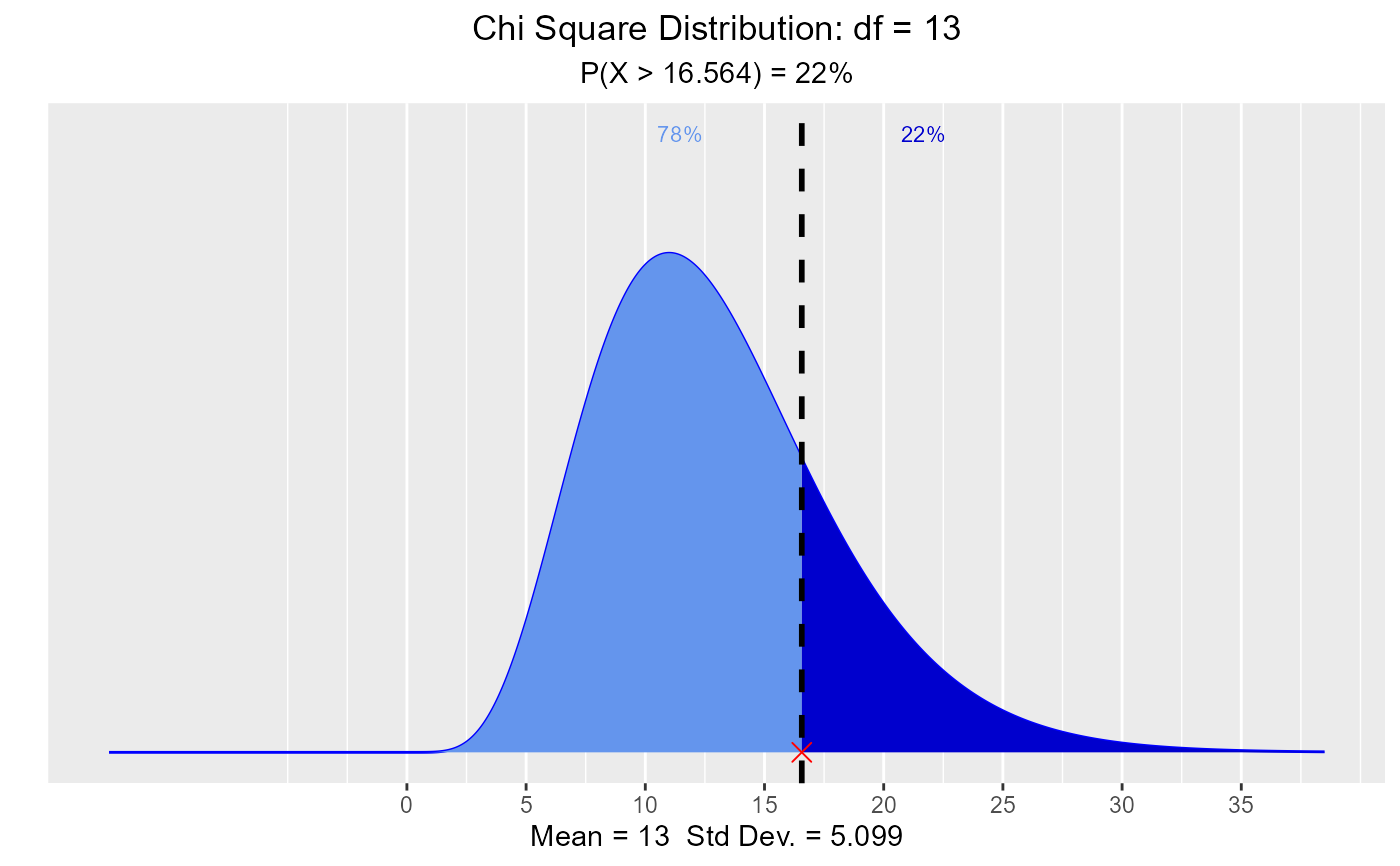# visualize probability from a given quantile.
vdist_chisquare_prob(13.58, 11, 'lower')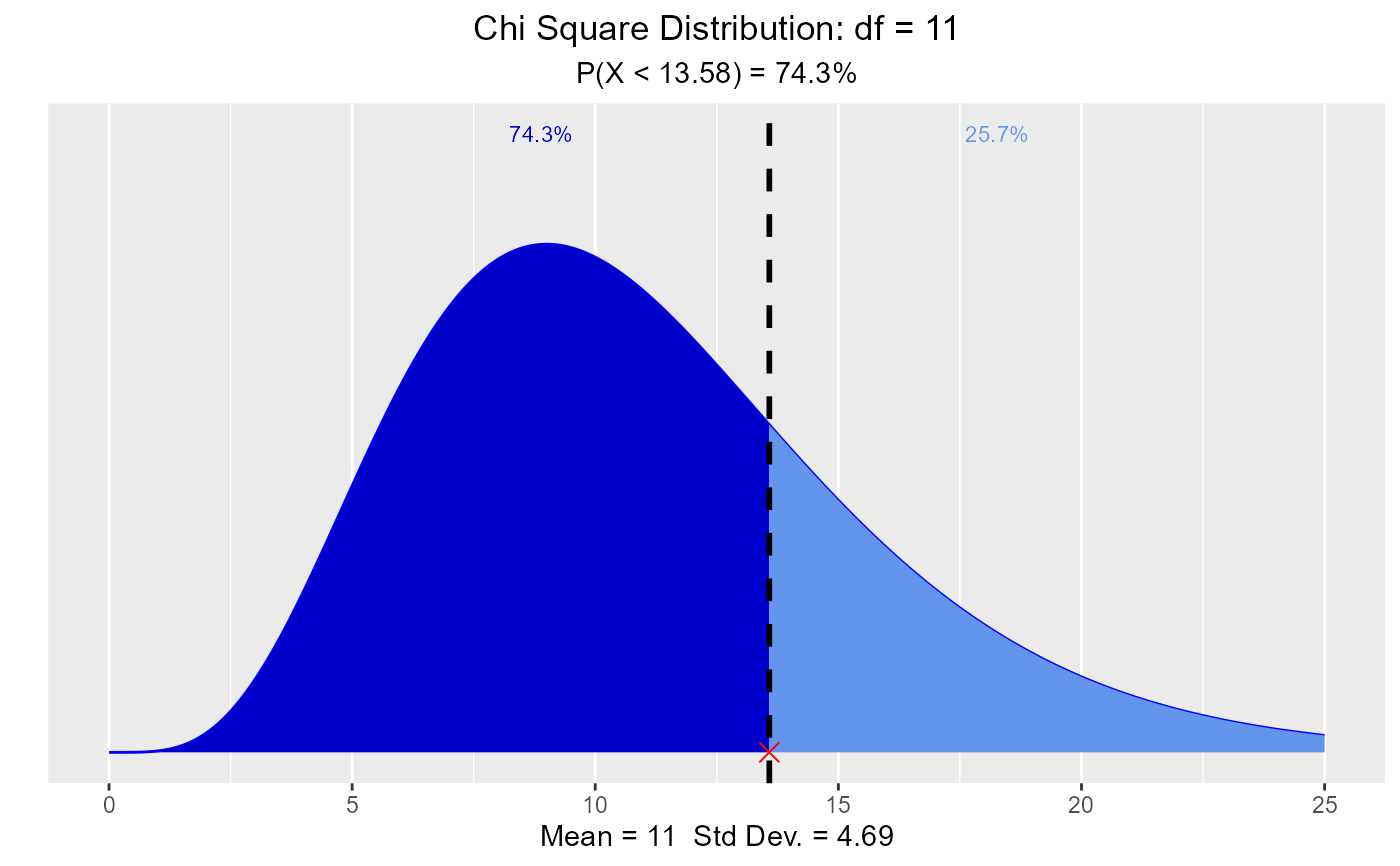vdist_chisquare_prob(15.72, 13, 'upper')